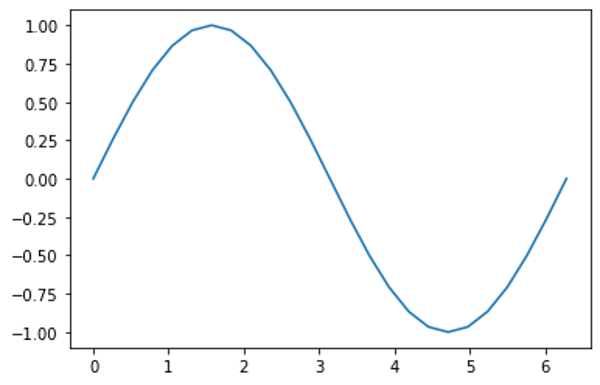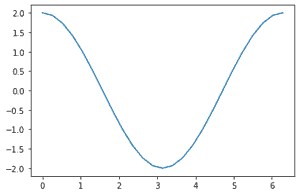Related Tags

python
pytorch
deep learning

### Overview

Autograd is an automatic differentiation package in the PyTorch library that helps train a neural network through graph computing. Instead of executing instructions immediately (also known as eager execution), Autograd builds a graph and uses it to speed up the calculation of derivatives, which are needed for training a neural network.

### How it works

When training neural networks, weights and biases must be adjusted during backpropagation. This is done by finding the gradient of every output for every input. For an input vector x of n dimensions and an output vector of m dimensions, the matrix of these gradients would be:

This matrix, denoted by J, is called a Jacobian. Mathematically, Autograd calculates the Jacobianvector products.

For example, let's suppose we have another vector, v, and v is the gradient of the following scalar function:

$l$ = g(y): $v=\left(\begin{array}{ccc}\frac{\partial l}{\partial y_{1}} & \cdots & \frac{\partial l}{\partial y_{m}}\end{array}\right)^{T}$

Then, using the chain rule, the Jacobian-vector product gives a gradient l for x, as follows:

Recall that the chain rule is as follows:Chain rule

In neural networks, the partial derivatives of the model’s outputs for its inputs would need multiple local partial derivatives for each multiplied learning weight, every activation function, and so on. This is computationally very expensive.

Autograd assists in solving this problem. It tracks all the operations performed on a tensor (vector) and stores them in the tensor itself. The tensor has a graph of its own that is made with the operations performed on it. This speeds up the process of derivative calculations.

### Example

In the following code, we import the torch package for Autograd, and the matplotlib package for plotting graphs:

import torch
import matplotlib.pyplot as plt
import matplotlib.ticker as ticker
import math
Import the required modules

Next, we create a one-dimensional tensor with the flag requires_grad=True:

StartVector = torch.linspace(0., 2. * math.pi, steps=25, requires_grad=True)
print(StartVector)
Create a one-dimensional tensor

Here is the output:

tensor([0.0000, 0.2618, 0.5236, 0.7854, 1.0472, 1.3090, 1.5708, 1.8326, 2.0944,
2.3562, 2.6180, 2.8798, 3.1416, 3.4034, 3.6652, 3.9270, 4.1888, 4.4506,
4.7124, 4.9742, 5.2360, 5.4978, 5.7596, 6.0214, 6.2832],
requires_grad=True)

Then, we perform the sin operation on the tensor and plot it. We use the .detach() method to stop tracking the operation so that it doesn't get included in the graph being built.

b = torch.sin(StartingVector)
plt.plot(StartingVector.detach(), b.detach())
Plot the graph of the sin operation on the StartingVector tensor

Here is the output:The graph of the sin operation performed on StartingVector

As you can see, b is the sine of StartingVector. If we print b, we see that it has the history of its operations in it:

print(b)

Here is the output:

tensor([ 0.0000e+00,  2.5882e-01,  5.0000e-01,  7.0711e-01,  8.6603e-01,
9.6593e-01,  1.0000e+00,  9.6593e-01,  8.6603e-01,  7.0711e-01,
5.0000e-01,  2.5882e-01, -8.7423e-08, -2.5882e-01, -5.0000e-01,
-7.0711e-01, -8.6603e-01, -9.6593e-01, -1.0000e+00, -9.6593e-01,
-8.6603e-01, -7.0711e-01, -5.0000e-01, -2.5882e-01,  1.7485e-07],
grad_fn=<SinBackward>)

The .grad_fn attribute contains information about the last operation. In this case, that operation is the sin operation.

Similarly, we can view the history of other operations:

c = 2 * b
print(c)

d = c + 1
print(d)

out = d.sum()
print(out)
Perform other operations to view their history

Here is the output:

tensor([ 0.0000e+00,  5.1764e-01,  1.0000e+00,  1.4142e+00,  1.7321e+00,
1.9319e+00,  2.0000e+00,  1.9319e+00,  1.7321e+00,  1.4142e+00,
1.0000e+00,  5.1764e-01, -1.7485e-07, -5.1764e-01, -1.0000e+00,
-1.4142e+00, -1.7321e+00, -1.9319e+00, -2.0000e+00, -1.9319e+00,
-1.7321e+00, -1.4142e+00, -1.0000e+00, -5.1764e-01,  3.4969e-07],

tensor([ 1.0000e+00,  1.5176e+00,  2.0000e+00,  2.4142e+00,  2.7321e+00,
2.9319e+00,  3.0000e+00,  2.9319e+00,  2.7321e+00,  2.4142e+00,
2.0000e+00,  1.5176e+00,  1.0000e+00,  4.8236e-01, -3.5763e-07,
-4.1421e-01, -7.3205e-01, -9.3185e-01, -1.0000e+00, -9.3185e-01,
-7.3205e-01, -4.1421e-01,  4.7684e-07,  4.8236e-01,  1.0000e+00],

tensor(25.0000, grad_fn=<SumBackward0>)

As seen above, c, d , and out have their operation information stored in grad_fn.

When computing derivatives, the loss function has a single value, so the out tensor also has only one value, 25.000, obtained by summing d.

We can view all past operations on d by using the grad_fn.next_functions method:

print(d.grad_fn)
print(d.grad_fn.next_functions.next_functions.next_functions.next_functions)
View past operations on the tensor, d

Here is the output:

<AddBackward0 object at 0x7fa00048dfd0>
((<MulBackward0 object at 0x7fa00048d3a0>, 0), (None, 0))
((<SinBackward object at 0x7fa00048dfd0>, 0), (None, 0))
()

To get the gradients, we use the .backwards() method:

out.backward()
plt.plot(StartingVector.detach(), StartingVector.grad.detach())
Get the gradients and plot the graph of the differentiated function

Here is the output:

tensor([ 2.0000e+00,  1.9319e+00,  1.7321e+00,  1.4142e+00,  1.0000e+00,
5.1764e-01, -8.7423e-08, -5.1764e-01, -1.0000e+00, -1.4142e+00,
-1.7321e+00, -1.9319e+00, -2.0000e+00, -1.9319e+00, -1.7321e+00,
-1.4142e+00, -1.0000e+00, -5.1764e-01,  2.3850e-08,  5.1764e-01,
1.0000e+00,  1.4142e+00,  1.7321e+00,  1.9319e+00,  2.0000e+00])The graph of the differentiated function 2 * sin(StartingVector) + 1

The graph gives us the value obtained by differentiating 2*sin(StartingVector) + 1, which is the operation performed on the input, from StartingVector.

Note: The gradients (.grad ) are only stored in leaf Nodes, that is, the input vectors. In this case, the input vector was StartingVector. Therefore, c.grad, d.grad, and so on will give None.

When declaring Tensors for models using torch, requires_grad is assumed to be set toTrue. There are two ways of disabling this:

• Directly set the flag to False
• Use torch.no_grad
a = torch.ones(2, 3, requires_grad=True)
b = 2 * a

c = a + b

In such cases where Autograd is not enabled, the torch.enable_grad() method is used.

### Pros and cons of using Autograd

Autograd runs code in graph execution mode as opposed to eager execution.

• Since eager execution runs all operations one by one, it cannot take advantage of potential acceleration resources. Graph execution extracts tensor computations from Python and builds an efficient graph before evaluation.
• It allows for better parallel computing, since Autograd allocates resources more efficiently to run multiple operations in parallel. This also results in better utilization of GPUs or TPUs.

• Autograd is unsuitable for smaller applications since it takes initial computing power to construct a graph.
• Depending on the implementation, the program can also become more complex.

RELATED TAGS

python
pytorch
deep learning

CONTRIBUTOR